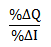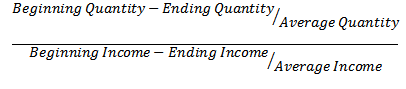Calculating the income elasticity of demand - FreeEconHelp.com, Learning Economics... Solved!

## 5/30/11

Calculating the income elasticity of demand is actually very easy.  You just need to know several of the values, then plug them into their proper place.Below is the equation for the income elasticity of demand.  In order to calculate this, we need the beginning and ending income, and the beginning and ending quantity.  By beginning and ending, I mean before and after the income/quantity change.Here just plug in the values given to their appropriate spot.  Some students get confused about which income/quantity goes first, but actually it does not matter as long as you are consistent.  Try it with some example values and you will see that subtracting ending from beginning or vice verse has no impact on the final answer.